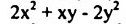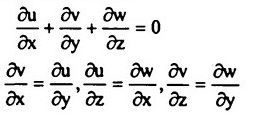top of pageSearch

# Fluid Mechanics (341-360)

Updated: Apr 23, 2020

341. The standard step method of gradually varied flow computations involves the

application of continuity and energy equations

342.A. Positive surge travelling upstream-Occurs on upstream of gate that is partly closed suddenly B. Positive surge travelling downstream-Occurs on downstream of gate that is partly open suddenly C. Negative surge travelling upstream-Occurs on upstream of gate that is partly open suddenly D. Negative surge travelling downstream-Occurs on downstream of gate that is partly closed suddenly

343.relate to water surface profiles in gradually varied flow of an open channel.

M and S curves meet y line asymptotically and tend to be horizontal as y -

C and C curves will be slightly curved if Chezy's equation is used.

Otherwise, they tend to be straight lines.

344. In a supersonic flow, a diverging passage results in decrease in pressure and density

345. In a normal shock wave in a one dimensional flow, the

pressure,density and temperature increases

346. A three-dimensional state of stress can be split into the two components, the

hydrostatic state and the deviatoric component. Then magnitude of the

hydrostatic state pressure is given by347. In a two-dimensional incompressible flow, if the fluid velocity components are

given by u = x - 4y, v = -y - 4x then the stream function ψ is given by348. Which of the following equations will be satisfied by irrotational • flow of ~an

incompressible fluid ?349. A circular surface area 200 cm² is inclined to the x and y axes such that the unit vector normal to the surface is given by n = 0.4i + 0.3j. If a force of 2000 kg acts on the x-axis, then the normal stress on the surface will be

5 kg/cm²

350. Given the x-component of the velocity u = 6xy - 2x2, the y-component of the

flow v is given by 4xy-3y²

351. A jet of water issues from a 5 cm diameter nozzle, held vertically upwards, at a

velocity of 20 m/sec. If air resistance consumes 10% of the initial energy of the

jet, then it would reach a height; above the nozzle, of 18.35 m

352. The ratio of pressures between two points A and B located respectively at depths 0.5 m and 2 m below a constant level of water in a tank is 1:4

353.A wooden plank (sp. gr. 0.5) 1 m x lm x 0.5 m floats in water with 1.5 kN load on

it with 1 m x 1 m surface horizontal. The depth of plank lying below water surface

shall be 0.403 m

354.Which of the following assumptions are made in the analysis of jet impinging

normally on a moving plate ?

1. Friction between jet and plate is neglected.

3. Plate moves at a constant velocity.

355.A Rankine (oval) half body PP is subjected to a two dimensional flow (x, y

coordinate directions from origin O) with uniform velocity V, resulting in typical

streamlines as shown in Fig by dotted linesThe point A on the body surface is

stagnation point

356357. The head loss in a pipe of diameter d, carrying oil at a flow rate Q over a

distance 1 is h. The pipe is replaced by another with half the diameter, all other

things remaining the same. The head loss in this case will be 32.0 h

358359. The discharge per metre width at the foot of a spillway is 10 m3/s at a velocity of 20 m/s. A perfect free hydraulic jump will occur at the foot of the spillway when the tail water depth is approximately equal to 6.50 m

360. The differential equation for energy for a reversible adiabatic flow may take the form